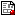[Previous] [Next] [Up] [Top]

# Assignment 4

Multivariate Data Analysis
Psychology 6140

## Supplements

Interactive Rank Exercise
Here you get to try out your understanding of the rank of a matrix online! Try several exercises at difficulty level 1, then try several at level 2. Highly recommended.
Linear Transformations Applet
Enter coefficients for a 2 × 2 matrix, and see the effect it has as a linear transformation.
Linear transformations of points Another online linear transformation applet.
imlproj.sasProjections: Projecting a vector in a vector space, and the Gram-Schmidt process, in excruciating detail!

## Problems (due: next week)

From the Matrix Algebra Problems (MAP) handout: Try using my SAS IML matrix library or R to do the following problems from Green and Carroll. For SAS, start your session with
```ods listing;
proc iml;
%include iml(matlib);
reset print log;
```
which defines functions proj(y,X) (project y on X), R(X) (rank of matrix X) among others.

For R, there is a similar collection of functions in the matlib package you can load into your R session.

```library(matlib)
```

• Chap 3, Problem 7 -- part (a) only. For this problem, try to follow G&C's approach or that in the IML example imlproj. You can create a matrix X containing all three vectors (as columns) with the expression
```X = t({ 1 2 3,  3 0 2, 3 1 1});
```
You can use the gsorth function to verify your results.
• Chap 4, Problem 7. For this problem, you can use the R(X) function to verify your answer, but use some other method to find the rank.
• Chap 4, Problem 9b